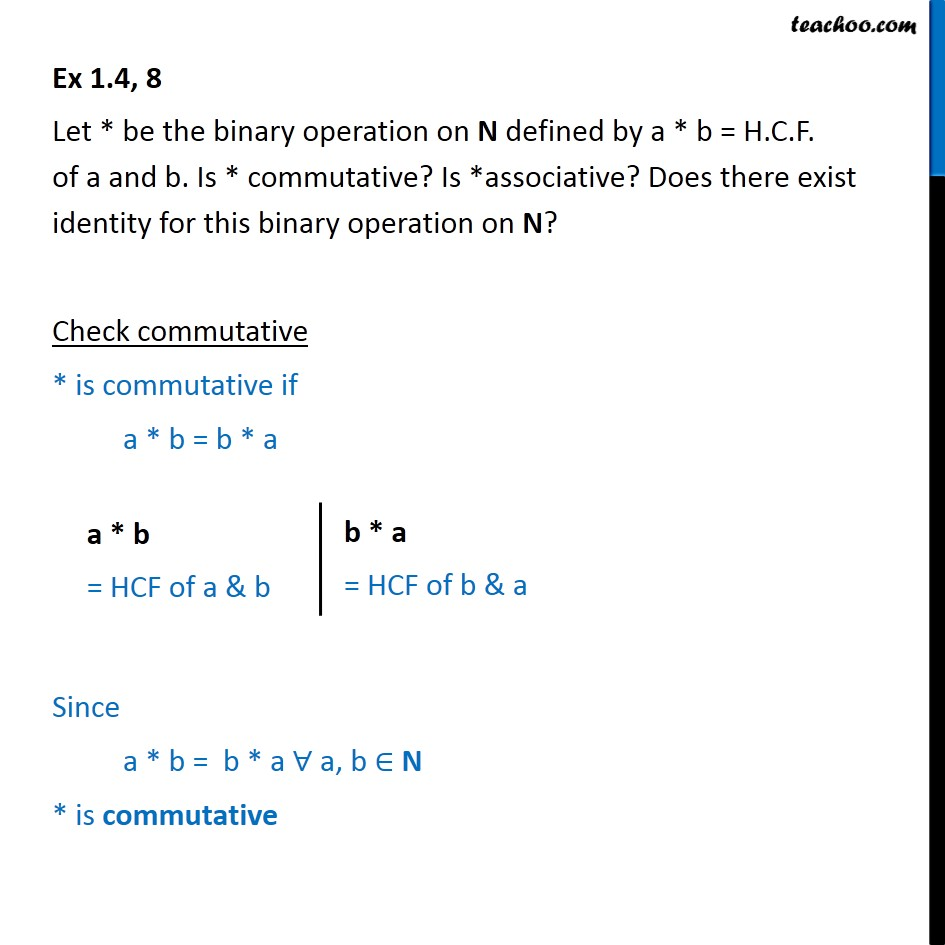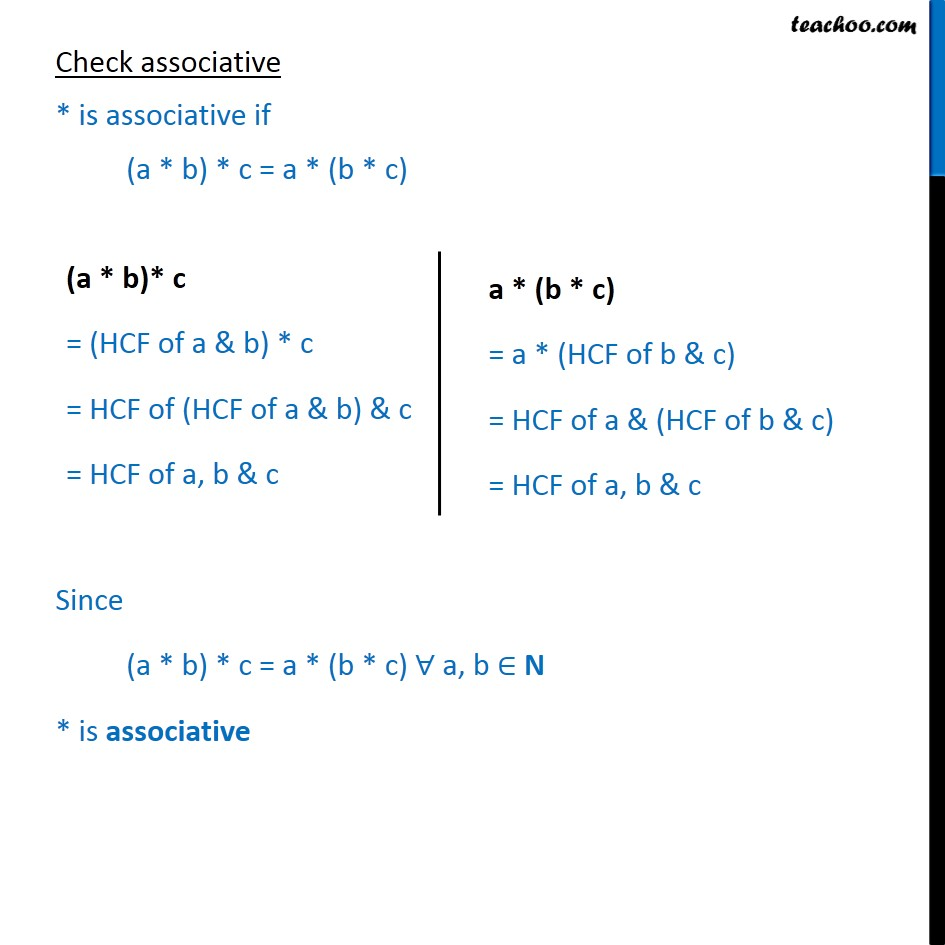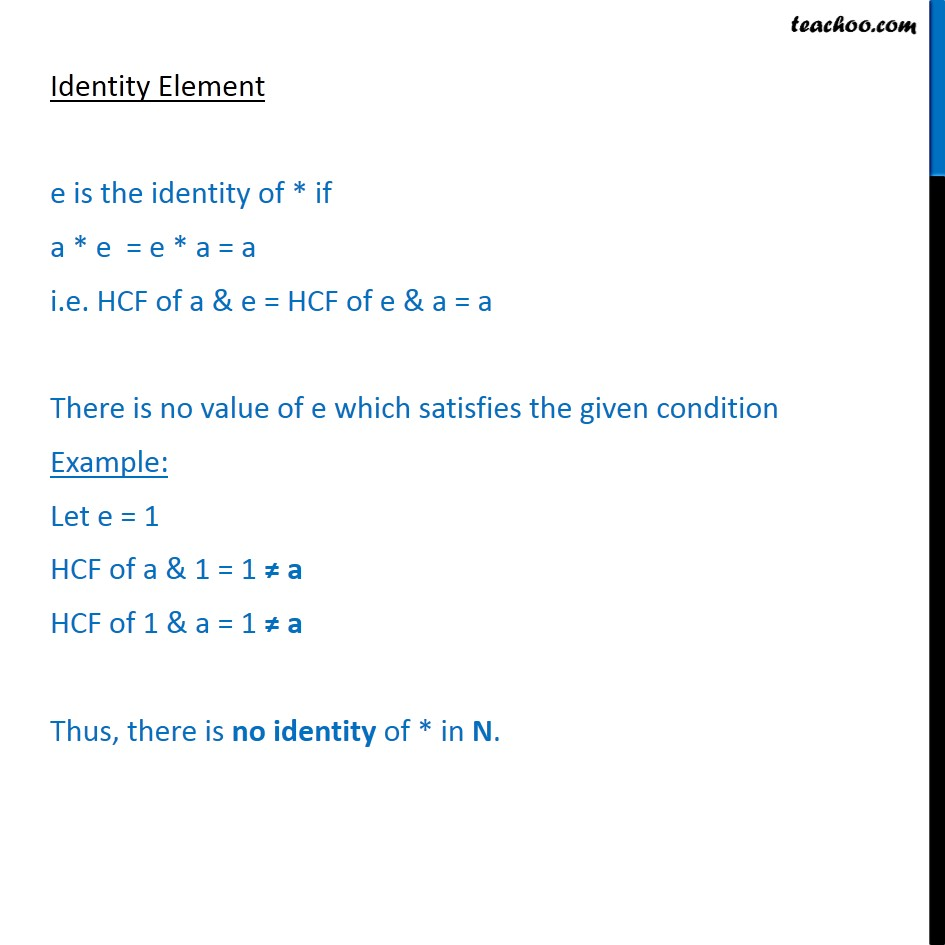Ex 1.4

Chapter 1 Class 12 Relation and Functions (Term 1)
Serial order wise### Transcript

Ex 1.4, 8 Let * be the binary operation on N defined by a * b = H.C.F. of a and b. Is * commutative? Is *associative? Does there exist identity for this binary operation on N? Check commutative * is commutative if a * b = b * a Since a * b = b * a ∀ a, b ∈ N * is commutative Check associative * is associative if (a * b) * c = a * (b * c) Since (a * b) * c = a * (b * c) ∀ a, b ∈ N * is associative Identity Element e is the identity of * if a * e = e * a = a i.e. HCF of a & e = HCF of e & a = a There is no value of e which satisfies the given condition Example: Let e = 1 HCF of a & 1 = 1 ≠ a HCF of 1 & a = 1 ≠ a Thus, there is no identity of * in N.